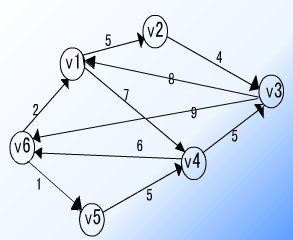#include <stdio.h> #include <string.h> #include <iostream> #include <algorithm> using namespace std; int n = 0; int a; int menu() { int m; printf(" 求最小生成树\n"); printf(" ________________________________\n\n"); printf(" 1 输入城市之间的信息\n"); printf(" 2 判断是否能构成一个最小生成树\n"); printf(" 3 查询信息\n"); printf(" 4 退出\n"); printf(" ________________________________\n\n"); printf(" 请输入所选功能1--4\n"); scanf("%d",&m); return m; } void xin_input() { printf("1 遍历所有城市的最小生成树\n"); printf("2 查询两个城市之间的距离\n"); printf("3 退出\n"); //printf("________________________________\n\n"); } //以下为克鲁斯卡尔算法 typedef struct node //构造一个结构体，两个城市可以看成起点和终点，之间的路道可以看成一个边 { int st; //起点 int ed; //终点 int dis;//距离 }node; node p; int pre,rank; bool cmp(node p1,node p2) { return p1.dis < p2.dis; } //下面三个函数是并查集，其作用是检验当一条边添加进去，是否会产生回路 void set(int x) { pre[x] = x;//初始化 rank[x] = 0; } int find(int x)//找到这个点的祖先 { if(x != pre[x]) pre[x] = find(pre[x]); return pre[x]; } void Union(int x,int y)//将这两个添加到一个集合里去 { x = find(x); y = find(y); if(rank[x] >= rank[y]) { pre[y] = x; rank[x] ++; } else pre[y] = x; } void Kruskal() // { int ans = 0,i,j,k = 0; for(i = 1;i <= n;i ++) set(i); for(i = 1;i <= n;i ++) for(j = i + 1;j <= n;j ++) { p[++k].st = i; p[k].ed = j; p[k].dis = a[i][j]; //先把所有城市之间的路段看成一个边 } sort(p + 1,p + k + 1,cmp);//把所有的边按从小到大进行排序 int count = 0; for(i = 1;i <= k;i ++) { if(find(p[i].st) != find(p[i].ed))//如果这两点连接在一起不构成一个回路，则执行下面操作 { printf("第%d条路段为：%d--%d,权值为%d\n",++ count,p[i].st,p[i].ed,p[i].dis);//将这条边的起点、终点打印出来 ans += p[i].dis; //说明这条路段要用 Union(p[i].st,p[i].ed); } } printf("遍历所有城市的最短路程为: %d\n",ans);//最短遍历的路程 //printf("________________________________\n"); } //普里姆算法 int prim() { int close,low,i,j,ans = 0;//close[j]表示离j最近的顶点，low[j]表示离j最短的距离 bool use; use = 1; for(i = 2;i <= n;i ++) { low[i] = a[i]; //初始化 close[i] = 1; use[i] = 0; } for(i = 1;i < n;i ++) { int min = 100000000,k = 0; for(j = 2;j <= n;j ++) { if(use[j] == 0 && min > low[j])//找到最小的low[]值，并记录 { min = low[j]; k = j; } } //printf("%d %d\n",close[k],k); for(j = 2;j <= n;j ++) { if(use[j] == 0 && low[j] > a[k][j]) { low[j] = a[k][j]; //修改low[]值和close[]值 close[j] = k; } } ans += a[close[k]][k]; } return ans; } //求最短路径-单源点到其余点的最短路径(Dijkstra算法) void ShortestPath_DIJ(int x,int y) //X指单源点，y指目标点 { int i,d,j; bool use; for(i = 1;i <= n;i ++) { use[i] = 0; //初始化 d[i] = a[x][i]; } use[x] = 1;//这个点肯定用过 d[x] = 0; for(i = 1;i < n;i ++)//执行n-1次操作 { int min = 100000000,v; for(j = 1;j <= n;j ++) { if(use[j] == 0 && min > d[j])//找出最短距离的 { min = d[j]; v = j; } } use[v] = 1; for(j = 1;j <= n;j ++) { if(use[j] == 0 && d[j] > min + a[v][j])//修改d[]的值 { d[j] = min + a[v][j]; } } } printf("%d城市到城市%d的最短距离为: %d\n",x,y,d[y]); } //求每一对顶点之间的最短路径(Floyd算法) int b; void ShortestPath_FLOYD() //这个算法耗时多(n^3),不过容易理解 { int i,j,k; for(i = 1;i <= n;i ++) for(j = 1;j <= n;j ++) b[i][j] = a[i][j]; //先b[][]进行保存，避免a[][]的值发生变化 for(k = 1;k <= n;k ++) for(i = 1;i <= n;i ++) for(j = 1;j <= n;j ++) if(b[i][j] > b[i][k] + b[k][j]) b[i][j] = b[i][k] + b[k][j]; while(1) { int st,ed; printf("请输入两个城市的编号:\n");//对此进行多次操作 scanf("%d%d",&st,&ed); printf("%d 城市到 %d 城市的最短距离为: %d\n",st,ed,b[st][ed]); //printf("________________________________\n"); char str; printf("是否要继续? yes or no\n"); scanf("%s",str); if(strcmp(str,"no") == 0) { //printf("________________________________\n"); break; } } } void shuru()//输入城市信息 { int i,j; if(n != 0) { char str; printf("是否要确定输入新的城市之间的信息? yes or no ?\n"); scanf("%s",str); if(strcmp(str,"no") == 0) { return ; } } printf("请输入城市的个数:\n"); scanf("%d",&n); printf("输入%d个城市的邻接矩阵:\n",n); for(i = 1;i <= n;i ++) { for(j = 1;j <= n;j ++) scanf("%d",&a[i][j]); } //printf("________________________________\n"); } void chaxun() //一个查徇两个城市最短径的操作 { while(1) { int t1,t2; printf("输入第一个城市的编号:\n"); scanf("%d",&t1); printf("输入第二个城市的编号:\n"); scanf("%d",&t2); ShortestPath_DIJ(t1,t2);//调用 char str; printf("是否要继续? yes or no\n"); scanf("%s",str); if(strcmp(str,"no") == 0) { //printf("________________________________\n"); break; } } } void chaxun_func() { if(n == 0) { printf("这里没有城市之间的信息\n"); return; } xin_input(); printf("请选择以上功能:1-3\n"); int m1; scanf("%d",&m1); printf("\n"); if(m1 == 1) Kruskal(); else if(m1 == 2) { chaxun(); } //else // break; } void judge() { int ans; ans = prim(); if(ans >= 100000000) printf("不能构成最小生成树\n"); else printf("能构成最小生成树\n"); } int main() { while(1) { switch(menu()) { case 1:shuru();break; case 2:judge();break; case 3: chaxun_func();break; case 4:goto loop; } } loop:; return 0; }

matlab 蚁群算法报错 小白求助
function [R_best,L_best,L_ave,Shortest_Route,Shortest_Length]=ACATSP(C,NC_max,m,Alpha,Beta,Rho,Q) %%------------------------------------------------------------------------- %% 主要符号说明 %% C n个城市的坐标，n×2的矩阵 load C.mat % 39个城市问题 %% NC_max 最大迭代次数 NC_max = 30; %% m 蚂蚁个数 m = 20; %% Alpha 表征信息素重要程度的参数 Alpha = 0.3; %% Beta 表征启发式因子重要程度的参数 Beta = 0.4; %% Rho 信息素蒸发系数 Rho = 0.5; %% Q 信息素增加强度系数 Q = 0.4; %% R_best 各代最佳路线 %% L_best 各代最佳路线的长度 %%========================================================================= %%第一步：变量初始化 n=size(C,1);%n表示问题的规模（城市个数） D=zeros(n,n);%D表示完全图的赋权邻接矩阵 for i=1:n for j=1:n if i~=j D(i,j)=((C(i,1)-C(j,1))^2+(C(i,2)-C(j,2))^2)^0.5; else D(i,j)=eps; %i=j时不计算，应该为0，但后面的启发因子要取倒数，用eps（浮点相对精度）表示 end D(j,i)=D(i,j); %对称矩阵 end end Eta=1./D; %Eta为启发因子，这里设为距离的倒数 Tau=ones(n,n); %Tau为信息素矩阵 Tabu=zeros(m,n); %存储并记录路径的生成 NC=1; %迭代计数器，记录迭代次数 R_best=zeros(NC_max,n); %各代最佳路线 L_best=inf.*ones(NC_max,1); %各代最佳路线的长度 L_ave=zeros(NC_max,1); %各代路线的平均长度 while NC <=NC_max %停止条件之一：达到最大迭代次数，停止 %%第二步：将m只蚂蚁放到n个城市上 Randpos=[]; %随即存取 for i=1:(ceil(m/n)) Randpos=[Randpos,randperm(n)]; end Tabu(:,1)=(Randpos(1,1:m))'; %此句不太理解？ %%第三步：m只蚂蚁按概率函数选择下一座城市，完成各自的周游 for j=2:n %所在城市不计算 for i=1:m visited=Tabu(i,1:(j-1)); %记录已访问的城市，避免重复访问 J=zeros(1,(n-j+1)); %待访问的城市 P=J; %待访问城市的选择概率分布 Jc=1; for k=1:n if length(find(visited==k))==0 %开始时置0 J(Jc)=k; Jc=Jc+1; %访问的城市个数自加1 end end %下面计算待选城市的概率分布 for k=1:length(J) P(k)=(Tau(visited(end),J(k))^Alpha)*(Eta(visited(end),J(k))^Beta); end P=P/(sum(P)); %按概率原则选取下一个城市 Pcum=cumsum(P); %cumsum，元素累加即求和 Select=find(Pcum>=rand) %若计算的概率大于原来的就选择这条路线 to_visit=J(Select(1)) Tabu(i,j)=to_visit; end end if NC>=2 Tabu(1,:)=R_best(NC-1,:); end %%第四步：记录本次迭代最佳路线 L=zeros(m,1); %开始距离为0，m*1的列向量 for i=1:m R=Tabu(i,:); for j=1:(n-1) L(i)=L(i)+D(R(j),R(j+1)); %原距离加上第j个城市到第j+1个城市的距离 end L(i)=L(i)+D(R(1),R(n)); %一轮下来后走过的距离 end L_best(NC)=min(L); %最佳距离取最小 pos=find(L==L_best(NC)); R_best(NC,:)=Tabu(pos(1),:); %此轮迭代后的最佳路线 L_ave(NC)=mean(L); %此轮迭代后的平均距离 NC=NC+1 ; %迭代继续 %%第五步：更新信息素 Delta_Tau=zeros(n,n); %开始时信息素为n*n的0矩阵 for i=1:m for j=1:(n-1) Delta_Tau(Tabu(i,j),Tabu(i,j+1))=Delta_Tau(Tabu(i,j),Tabu(i,j+1))+Q/L(i); %此次循环在路径（i，j）上的信息素增量 end Delta_Tau(Tabu(i,n),Tabu(i,1))=Delta_Tau(Tabu(i,n),Tabu(i,1))+Q/L(i); %此次循环在整个路径上的信息素增量 end Tau=(1-Rho).*Tau+Delta_Tau; %考虑信息素挥发，更新后的信息素 %%第六步：禁忌表清零 Tabu=zeros(m,n); %%直到最大迭代次数 end %%第七步：输出结果 Pos=find(L_best==min(L_best)); %找到最佳路径（非0为真） Shortest_Route=R_best(Pos(1),:) %最大迭代次数后最佳路径 Shortest_Length=L_best(Pos(1)) %最大迭代次数后最短距离 subplot(1,2,1) %绘制第一个子图形 DrawRoute(C,Shortest_Route) %画路线图的子函数 subplot(1,2,2) %绘制第二个子图形 plot(L_best) hold on %保持图形 plot(L_ave,'r') title('平均距离和最短距离') %标题 function DrawRoute(C,R) %%========================================================================= %% DrawRoute.m %% 画路线图的子函数 %%------------------------------------------------------------------------- %% C Coordinate 节点坐标，由一个N×2的矩阵存储 %% R Route 路线 %%========================================================================= N=length(R); scatter(C(:,1),C(:,2)); hold on plot([C(R(1),1),C(R(N),1)],[C(R(1),2),C(R(N),2)],'g') hold on for ii=2:N plot([C(R(ii-1),1),C(R(ii),1)],[C(R(ii-1),2),C(R(ii),2)],'g') hold on end 最后报错。。。。 Index exceeds matrix dimensions. Error in ACATSP (line 127) to_visit=J(Select(1)); 求解答

Java学习的正确打开方式

Python——画一棵漂亮的樱花树（不同种樱花+玫瑰+圣诞树喔）

Python 植物大战僵尸代码实现(2):植物卡片选择和种植

2019年互联网寒冬，大批企业开始裁员，下图是网上流传的一张截图： 裁员不可避免，那如何才能做到不管大环境如何变化，自身不受影响呢？ 我们先来看一个有意思的故事，如果西游记取经团队需要裁员一名，会裁掉谁呢，为什么？ 西游记团队组成： 1.唐僧 作为团队teamleader，有很坚韧的品性和极高的原则性，不达目的不罢休，遇到任何问题，都没有退缩过，又很得上司支持和赏识(直接得到唐太宗的任命，既给袈...
shell脚本：备份数据库、代码上线

IntelliJ IDEA 简称 IDEA，被业界公认为最好的 Java 集成开发工具，尤其在智能代码助手、代码自动提示、代码重构、代码版本管理(Git、SVN、Maven)、单元测试、代码分析等方面有着亮眼的发挥。IDEA 产于捷克，开发人员以严谨著称的东欧程序员为主。IDEA 分为社区版和付费版两个版本。 我呢，一直是 Eclipse 的忠实粉丝，差不多十年的老用户了。很早就接触到了 IDEA...

Redis 面试题 1、什么是 Redis?. 2、Redis 的数据类型？ 3、使用 Redis 有哪些好处？ 4、Redis 相比 Memcached 有哪些优势？ 5、Memcache 与 Redis 的区别都有哪些？ 6、Redis 是单进程单线程的？ 7、一个字符串类型的值能存储最大容量是多少？ 8、Redis 的持久化机制是什么？各自的优缺点？ 9、Redis 常见性...

【超详细分析】关于三次握手与四次挥手面试官想考我们什么？

2019年Spring Boot面试都问了什么？快看看这22道面试题！
Spring Boot 面试题 1、什么是 Spring Boot？ 2、Spring Boot 有哪些优点？ 3、什么是 JavaConfig？ 4、如何重新加载 Spring Boot 上的更改，而无需重新启动服务器？ 5、Spring Boot 中的监视器是什么？ 6、如何在 Spring Boot 中禁用 Actuator 端点安全性？ 7、如何在自定义端口上运行 Sprin...
【图解】记一次手撕算法面试：字节跳动的面试官把我四连击了

【图解算法面试】记一次面试：说说游戏中的敏感词过滤是如何实现的？

GitHub 标星 1.6w+，我发现了一个宝藏项目，作为编程新手有福了！

Java知识体系最强总结(2020版)

2020年一线城市程序员工资大调查

Windows可谓是大多数人的生产力工具，集娱乐办公于一体，虽然在程序员这个群体中都说苹果是信仰，但是大部分不都是从Windows过来的，而且现在依然有很多的程序员用Windows。 所以，今天我就把我私藏的Windows必装的软件分享给大家，如果有一个你没有用过甚至没有听过，那你就赚了????，这可都是提升你幸福感的高效率生产力工具哦！ 走起！???? NO、1 ScreenToGif 屏幕，摄像头和白板...

2020年，冯唐49岁：我给20、30岁IT职场年轻人的建议

By 超神经场景描述：春节马上就要来临，在这个假期里，怎么能让自己放松，又不至于生疏了自己的老本行？不妨来玩一下编程向的小游戏吧，超神经在此整理了三款好玩有趣又有深度的游戏，快看看是不是...

CPU对每个程序员来说，是个既熟悉又陌生的东西？ 如果你只知道CPU是中央处理器的话，那可能对你并没有什么用，那么作为程序员的我们，必须要搞懂的就是CPU这家伙是如何运行的，尤其要搞懂它里面的寄存器是怎么一回事，因为这将让你从底层明白程序的运行机制。 随我一起，来好好认识下CPU这货吧 把CPU掰开来看 对于CPU来说，我们首先就要搞明白它是怎么回事，也就是它的内部构造，当然，CPU那么牛的一个东...
Python实战：抓肺炎疫情实时数据，画2019-nCoV疫情地图

B 站上有哪些很好的学习资源?